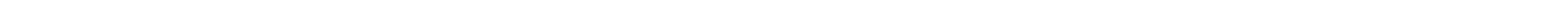Simple program to test whether the Labjack U3 is alive and connected
```########################## blinking_led.py ################################import timeimport u3d = u3.U3()while True:  d.toggleLED()  time.sleep(0.2)
```

The easyU3.py LIBRARY is designed to make it easy for students to use the LabJack A-to-D, D-to-A interface
```######################################### ezu3test.py ####################################  Test of Halverson's attempt at an easy interface to the LabJack U3 for student use.#  This is the 2nd version.  The 1st version was called easyU3test.py     2/1/2015import sysmypath=sys.pathif mypath.find('my_python') == -1:       #If true, then "my_python" could not be found  print "Sorry.  You need to be in my_python for Halverson's program to work."  print "(That''s because I am assuming that the tgraphlix.py and ezu3.py libraries are there.)"  exit()sys.path=mypath[0:mypath.find('my_python')]+'my_python'print "Use ^C to kill this program"import u3from ezu3 import *from time import sleepd = u3.U3() # Opens the first LabJackU3 found on the USBu3setup(d,['ain','ain','din','din','dout','dout','dout','dout'])blink_state=Truewhile True:   V0=ain0(d)       #Read analog inputs   V1=ain1(d)       #Analog inputs range from 0 to 2.4 Volts   B2=din2(d)      #Read digital inputs   B3=din3(d)   s=format(V0,"4.2f")               #Print the value of analog input 0   print "ain0=%s|" % s ,   for i in range(0,int(5*V0)):      #Print a bunch of spaces then a "0"      print " ",   print "0",   for i in range(0,13-int(5*V0)):   #Pad with blanks      print " ",   s=format(V1,"4.2f")               #Print the value of analog input 1   print "|ain1=%s|" % s ,   for i in range(0,int(5*V1)):      #Print a bunch of spaces then a "1"      print " ",   print "1",   for i in range(0,13-int(5*V1)):   #Pad with blanks      print " ",   print "|",   if not B2:      print "2   ",   else:      print "  2 ",   if not B3:      print "3  ",   else:      print "  3",   Temp=temperatureF(d)      s=format(Temp,"5.2f")               #Print the value of analog input 0   print "|Temp=%s F" % s   #Just for fun add blinking   LED(d,blink_state)   dout4(d,blink_state)   dout5(d,not blink_state)   dout6(d,blink_state)   dout7(d,not blink_state)   blink_state = not blink_state   dac0out(d,V0*2)                 #Send analog input 0 to DAC 0 after multiplying by 2   dac1out(d,V1*2)                 #Send analog input 1 to DAC 1 after multiplying by 2   sleep(0.25)                     #Wait for 1/4 second before looping again